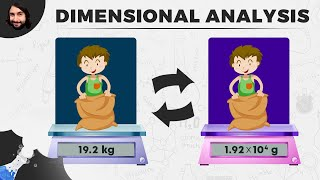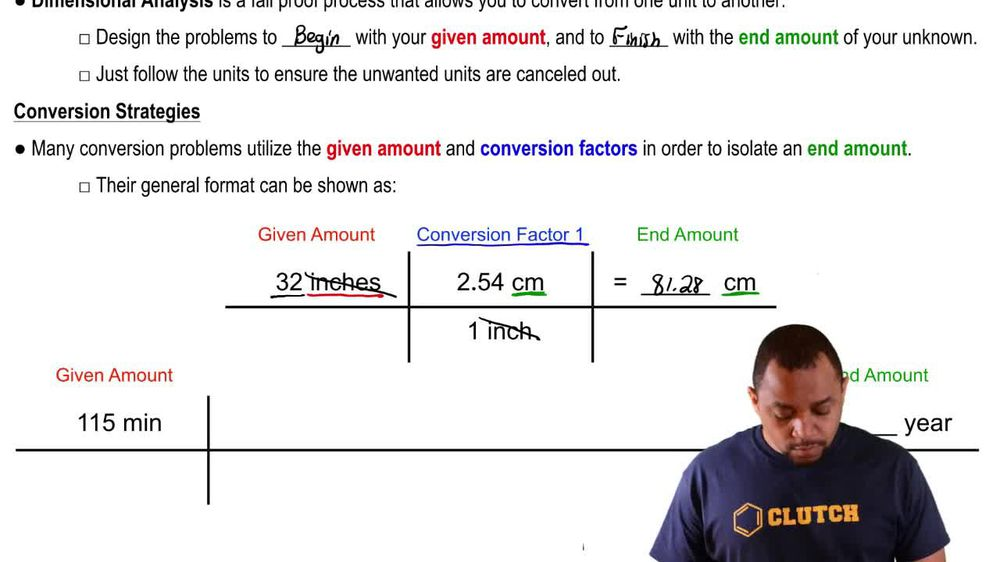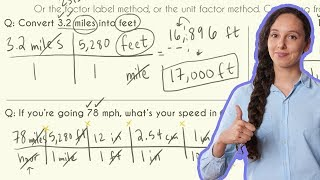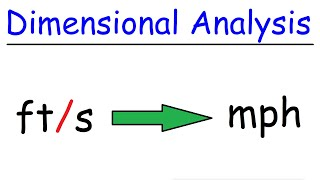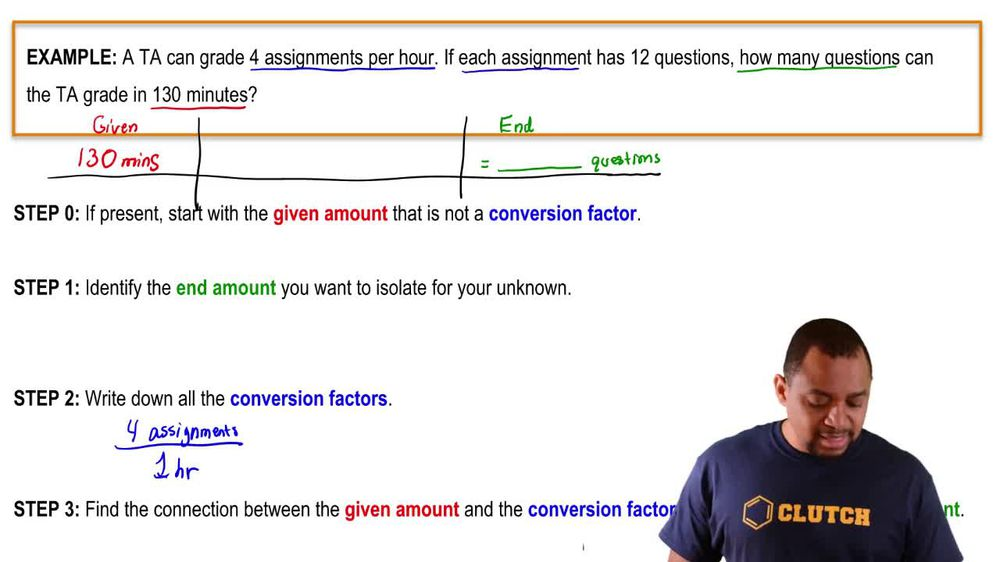Start typing, then use the up and down arrows to select an option from the list.
1. 1. Intro to General Chemistry2. Dimensional Analysis
Problem

# Perform the following conversions: (f) 0.02500 ft3 to cm3.

Relevant Solution1m
Play a video:
Hi everyone here we have a question telling us to convert 455 centimeters cubed, two inches cube. So we need to remember that one inch equals 2. cm so 400 55 centimeters cubed times one inch Over 2.54 cm. And this whole fraction is cubed and our centimeters cubed are canceling out, And that equals 0.8 inches cubed. And that is our final answer. Thank you for watching. Bye.